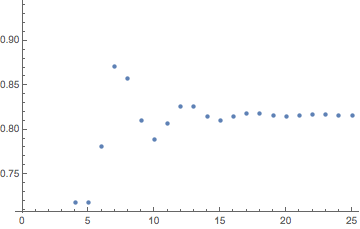# Closed form of :$\int_{-1}^1 x^{2k} (\operatorname{erf}(x))^k \,dx$ for $k$ is even integer and :$\int _{0}^{t}\exp(-x^2 \operatorname{erf}(x))dx$

This question is related to my question here such that i want to find a closed form of $\int_{-1}^1 x^{2k} (\operatorname{erf}(x))^k \,dx$ , for $k$ is even integer because for odd integer is $0$ as we have integrand of odd function , Really for $k=2$ it gives a nice closed form as shown here in wolfram alpha, Now my question here is :

Question:
Is it possible to get a Closed form of :$\int_{-1}^1 x^{2k} \operatorname{erf}(x)^k \,dx$ for $k$ is even integer?

Note: The Motivation of this question is to find a Series representation of :$\int _{0}^{t}\exp(-x^2 \operatorname{erf}(x))dx$

• As a general rule of thumb: since $1$ and $-1$ are not spacial points for the integrand, his definite integral is likely just as difficult as the indefinite integral. So: $\int x^4 \text{erf}(x)^2 dx$ has a known closed form, plug in and get your definite integral. But for $\int x^8 \text{erf}(x)^4 dx$ you are probably out of luck. – Gerald Edgar May 31 '18 at 23:57
• What for? Isn't it art for art's sake? – user64494 Jun 1 '18 at 9:03
• No, it is not art for art's sake. – Liviu Nicolaescu Jun 1 '18 at 13:15
• @Liviu Nikolaescu: Please, base your statement. – user64494 Jun 1 '18 at 17:50
• @user64494, Probably Liviu Nicolaescu think that is easy for evaluation – zeraoulia rafik Jun 1 '18 at 17:52

I understand from the OP that the motivation for this question is to find a series expansion in powers of $t$ of $$I(t)=\int _{0}^{t}\exp(-x^2 \operatorname{erf}(x))dx=\sum_{p=1}^\infty c_p t^p.$$ The coefficients $c_p=p^{-1}d_{p-1}$ follow from the series expansion $e^{-x^2\,{\rm erf}\,x}=\sum_{p=0}^\infty d_p x^p$, resulting in $$I(t)=\sum_{p=1}^\infty c_p t^p=t-\frac{t^4}{2 \sqrt{\pi }}+\frac{t^6}{9 \sqrt{\pi }}+\frac{2 t^7}{7 \pi }-\frac{t^8}{40 \sqrt{\pi }}-\frac{4 t^9}{27 \pi }+\frac{(\pi -28) t^{10}}{210 \pi ^{3/2}}+O\left(t^{11}\right).$$ The series $I(1)=\sum_{p=1}^\infty c_p$ seems to converge:Plot of $I_N=\sum_{p=0}^{N} c_p$ as a function of $N$ up to $N=25$. The value of $I_{25}=0.8162$ agrees with $I(1)=0.816377$ to three decimal places. For $N=50$ the agreement is up to six decimal places.
• @zeraouliarafik -- when I say $I_{50}=0.816377$ I am referring to the sum $I_{N}=\sum_{p=0}^N c_p$, which is the series expansion of $I(t)$ for $t=1$ to order 50; what you have evaluated with Wolfram alpha is $I(t)$ for $t=50$, which is a different thing. – Carlo Beenakker Jun 1 '18 at 18:08